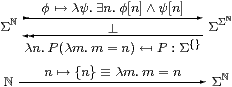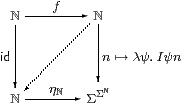## 10  Sobriety and description

In this section we prove that focus and description are inter-definable, for the natural numbers. In the following notation, we need to show that φ is a description iff P is prime.

Lemma 10.1 This is a retraction,cf. the connection between {} and η in [BW85, Lemma 5.1.3] and Lemma C 6.12.         ▫

[In fact there is an adjunction or coclosure as shown, since

 P ↦ φ≡λ n.P(λ m.n=m) ↦ λψ.∃ n.φ n∧ ψ n ≡ λψ.∃ n.P(λ m.n=m)∧ ψ n = λψ.∃ n.P(λ m.n=m∧ ψ n) ≤ λψ.P(λ m.ψ m) ≡  P

using the Euclidean principle (Remark 2.4) and the substitution rule for equality.]

First, we characterise the image of Σ↣ΣΣ.

Lemma 10.2 Γ⊢ P2ℕ is of the form λ ψ.∃ n.φ[n] ∧ ψ[n] for some Γ⊢φ:Σ iff it preserves ∃. In this case, φ=λ n.Pm.n=m).

Proof    Suppose that P preserves ∃. Then

 Pψ ⇔ P(λ m.∃ n.n=m∧ψ[n]) ⇔ ∃ n. P(λ m.n=m∧ψ[n]) ⇔ ∃ n. P(λ m.n=m)∧ψ[n]

by the Euclidean principle (Remark 2.4). The other way is easy.         ▫

For the rest of this section, P and φ will be related in this way.

Lemma 10.3 P preserves ⊤ iff φ satisfies the existence condition, and P preserves ∧ iff φ satisfies the uniqueness condition.

Proof    For the first, observe that P⊤ ⇔ ∃ n.φ[n]. For the second, if φ[n1]∧ψ1[n1] and φ[n2]∧ψ2[n2] then n1=n2=n by uniqueness.         ▫

Proposition 10.4 If P is prime then φ is a description, and the  n.φ[n] satisfies the rules for focusP (Definition 8.2).

Proof    As P is prime, it preserves ⊤, ∧ and ∃ because its double exponential transpose is a homomorphism, in particular with respect to ⊤, ∧ and ∃, as in Proposition 5.5. Also, P=λψ.∃ n.φ[n]∧ψ[n] by the Lemma, so Pψ⇔ψ[the  n.φ[n]], which means that the β-rules agree. For the η-rules,

φ=
 ⎧ ⎨ ⎩ n ⎫ ⎬ ⎭
≡(λ m.m=n)     iff     P=thunkn≡λψ.ψ[n],

which are respectively a description and prime, and then (the  m.φ[m])=(focusP)=n.         ▫

Corollary 10.5 Any overt discrete object that admits definition by description is sober. In particular, all sets and all objects of any topos are sober.         ▫

The converse is the case X=ℕ of Theorem 5.7, that if H→ΣU is a frame homomorphism (i.e. it preserves ⊤, ∧ and ∃) then it is an Eilenberg–Moore homomorphism. Of course, we showed that for LKLoc, by re-interpreting results from the literature, not for our abstract calculus. The proof below depends on (primitive) recursion, so only applies to ℕ rather than to discrete overt spaces in general, although when the whole theory is in place the result will hold for them too. [It is easy to show that if φ is a description then P preserves ⊤, ⊥, ∧ and ∨; then it is prime by Theorem G 10.2.]

But before considering ℕ we have to deal with 2≡{0,1}. As we did not ask for this as a base type in Section 2, ψ:Σ2 may be replaced by <ψ01>∈Σ×Σ, and similarly for the type of P. (Alternatively, one could formulate a result with ψ:Σ to capture the same point.) See B 11 for further discussion of disjoint unions in abstract Stone duality.

Proposition 10.6 Let α:Σ be decidable, with β⇔¬α (Definition 9.11). Then

 P ≡ λψ.(α∧ψ)∨(β∧ψ)

is prime. This justifies definition by cases:  (if α then 0 else 1)≡focusP.

Proof    Let Pαβ be the obvious generalisation, so

 γ∧ Pαβ = P(γ∧α)(γ∧β)

by distributivity. The equation that we have to prove for Definition 8.1 may be written

 (α∨β)∧F Pαβ  ⇔  (α∧F P⊤⊥) ∨ (β∧F P⊥⊤).

By the Euclidean principle (Remark 2.4) and the lattice laws, we have

 α∧F Pαβ ⇔ α∧F(α∧ Pαβ) ⇔ α∧F(α∧ Pα(α∧β)) ⇔ α∧F(α∧ Pα⊥) ⇔ α∧F(α∧ P⊤⊥) ⇔ α∧F P⊤⊥         ▫

Lemma 10.7 For any description φ, we define, by primitive recursion,

 φ≥≡⊤      φ>[n]≡φ≥[n+1]≡φ≥[n]∧¬φ[n]
 φ<≡⊥      φ≤[n]≡φ<[n+1]≡φ<[n]∨φ[n].

Then φ<[n] has the properties of the ordinary arithmetic order, that is, (the  m.φ[m])< n, and similarly for the others.         ▫

Proposition 10.8 If φ is a description then P is prime, and focusP satisfies the rules for the  n.φ[n].

Proof    The idea of the proof is to define a permutation f:ℕ≅ℕ that cycles the witness to 0 and any smaller values up by 1, leaving bigger numbers alone. The function f itself is defined by (cases and) primitive recursion, but it only has an inverse with general recursion. Let

f(n)  ≡

 0 if  φ[n] n if  φ<[n] n+1 if  φ>[n]
δ(n,m)  ≡

 m=0 ∧ φ[n] ∨ m=n ∧ φ<[n] ∨ m=n+1 ∧ φ>[n],

so δ(n,m) ⇔ f(n)=m. The operations Σf and I, defined by

 Σfθ ≡ λ n.∃ m.δ(m,n)∧θ[m] Iψ ≡ λ m.∃ n.δ(m,n)∧ψ[n],

are mutually inverse, because, by expanding disjunctions,

 ∃ m.δ(m,n) ⇔ φ[n]∨φ<[n]∨φ≥[n+1] ∃ n.δ(m,n) ⇔ (m=0∧∃ n.φ[n])∨(∃ n.m=n+1) δ(m,n1)∧δ(m,n2) ⇔ (n1=n2)∨(φ<[m]∧φ>[m]) δ(m1,n)∧δ(m2,n) ⇔ (m1=m2)∨(φ[n]∧φ<[n])∨ (φ[n]∧φ>[n+1]).

Thus Σf is a homomorphism, as is its inverse I by Proposition 3.5. But so too is evaluation at 0, so

 ψ ↦ θ ≡  ∃ n.δ(0,n) ≡ ∃ n.φ[n]∧ψ[n]

is a homomorphism, and P is prime. The β- and η-rules agree as before.         ▫

Remark 10.9 Sobriety says that the functor Σ(−):CopA in Definition 4.1 is full and faithful. But in this proof we only used the fact that it reflects invertibility, so it suffices to assume that ℕ is replete. Then f−1 is the diagonal mediator that is provided by the orthogonality property [Hyl91, Tay91].Corollary 10.10 H→ΣU is an Eilenberg–Moore homomorphism iff it preserves ⊤, ∧ and ∃.         ▫

This result can be extended from ℕ to higher types on the assumption of the continuity axiom (Remark 2.6). Hence there is a version of Theorem 5.7 that links the notions of homomorphism and sobriety that we have introduced entirely abstractly using the λ-calculus with those that arise from the ℕ-indexed lattice structure in Remarks 2.4ff. Although the proof would only require one more section, it begins to make serious use of domain-theoretic ideas, and so properly belongs in a discussion of that subject [F][G]. Besides, surprisingly much progress can be made with the development of topology without the extra axiom.